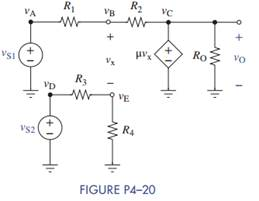### Create an Account

Home / Questions / Figure P4–20 is a dependent-source model of a subtractor Use MATLAB or node-voltage analys...

# Figure P4–20 is a dependent-source model of a subtractor Use MATLAB or node-voltage analysis to derive an exact expression for the output Then let μ ∞ and compare your answer to the expression

Figure P4–20 is a dependent-source model of a subtractor. Use MATLAB or node-voltage analysis to derive an exact expression for the output. Then let μ → ∞ and compare your answer to the expression for the subtractor in Eq. (4–32) in the text.Jun 15 2020 View more View LessSubscribe To Get Solution